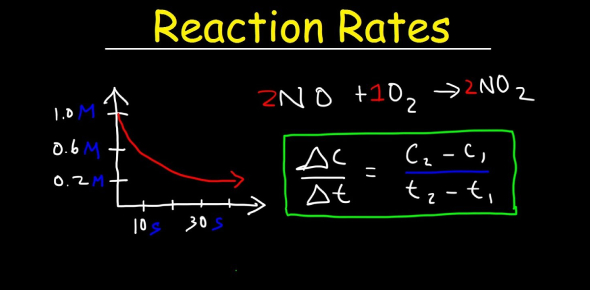# Rate Of Reaction Quiz! Trivia Test

10 Questions | Total Attempts: 1250SettingsThe rate of consequence is the speed at which a chemical reaction takes place. You may define the reaction rate as the speed at which reactants are converted into products. Reaction rates can vary dramatically. It is visible by watching the disappearance of a reactant or an object's appearance over time. Taking this quiz will help you see how much you know about reaction rates.

• 1.
Reactions eventually stop. What is generally the reason for this?
• A.

The catalyst has been used up

• B.

The particles have run out of energy

• C.

One or more of the reactants has been used up.

• 2.
Marble reacts with hydrochloric acid to produce calcium chloride, water, and carbon dioxide. In which of these mixtures is the rate of reaction likely to be the greatest?
• A.

1g of marble chips in 100cm3 of hydrochloric acid at 20°C

• B.

1g of powdered marble in 100cm3 of hydrochloric acid at 30°C.

• C.

1g of powdered marble in 100cm3 of hydrochloric acid at 20°C

• 3.
Manganese dioxide is a black powder that catalyzes the breakdown of hydrogen peroxide to water and oxygen. Which of the following statements is correct?
• A.

The mass of manganese dioxide will stay the same during the reaction

• B.

The catalysed reaction will produce more oxygen than the uncatalysed reaction

• C.

The particles in the catalysed reaction will have more energy than in the uncatalysed reaction

• 4.
Which of the following statements about collisions is correct?
• A.

All colliding particles have the same amount of energy

• B.

Only fast-moving particles collide with each other

• C.

Reactions can happen if the colliding particles have enough energy

• 5.
The rate of reaction increases as the temperature increases. Which of the following statements provides the best explanation for this?
• A.

At lower temperatures the particles do not collide with each other

• B.

At higher temperatures the particles have more energy, move faster and collide more often

• C.

Increasing the temperature increases the number of particles, so they collide more often

• 6.
A catalyst is best defined as a substance which increases the speed of a chemical reaction and which ___________ ______________ ______________.
• 7.
Which of the following industrial processes does not use a catalyst?
• A.

Oxidation of sulfur dioxide

• B.

Synthesis of ammonia

• C.

Electrolysis of sodium chloride

• 8.
When an excess of calcium carbonate reacts with dilute hydrochloric acid, the reaction becomes slower and finally stops because
• A.

The calcium carbonate is covered by bubbles of carbon dioxide

• B.

An insoluble layer of calcium chloride is formed

• C.

The calcium carbonate is all used up

• D.

The hydrochloric acid is all used up

• 9.
In each of four different experiments, the same mass of magnesium reacts with the same volume of excess sulfuric acid. Which set of conditions will result in the magnesium being used up the fastest?
• A.

Ribbon magnesium, 1 mol/dm3 of acid, 80 degrees celsius

• B.

Powdered magnesium, 0.5 mol/dm3 of acid, 20 degrees celsius

• C.

Ribbon magnesium, 0.5 mol/dm3 of acid, 80 degrees celsius

• D.

Powdered magnesium, 1 mol/dm3 of acid, 80 degrees celsius

• 10.
1. C(s) + CO2(g) ---> 2CO(g) 2. 2SO2(g) + O2(g) ---> 2SO3(g) 3. N2(g) + 3H2(g) ---> 2NH3 (g) 4. NaOH(aq) + HCl(aq) ---> NaCl(aq) + H2O(l) The rate of reaction 4 is least likely to get affected by pressure.
• A.

True

• B.

False

Related TopicsBack to top